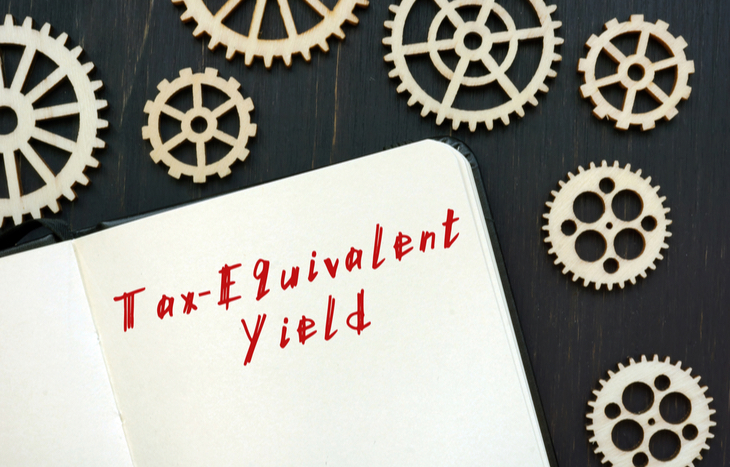Readers may hold bonds with different tax consequences. For instance, an investor or mutual fund may hold a municipal bond and a taxable corporate bond. Investors pay a different tax on the income from each. The various tax consequences of the two bonds make comparing the after-tax yield of the two pointless. This is where tax-equivalent yield comes in.

Paying taxes in income hurts the after-tax yield of a bond. Investors evaluating the effect that paying taxes has on the yield of their bonds need to make an apples-to-apples comparison. Additionally, this quick analysis will help them determine which yield will give them the best return after the investor pays taxes.

Going a bit further, the interest payments that you receive from the municipal bond are generally exempt from state and federal taxes. Therefore, the yield you see is the yield you get. On the other hand, the interest received from the taxable corporate bonds is taxable at the state and federal levels. So, investors need to adjust for taxes to make a fair comparison.

Keep in mind that taxes can be complicated. In addition to the tax rules for bond income, investors may pay different tax rates. Also, make sure the state you live in is not one of the few that impose taxes on municipal bond income. Please get in touch with your tax advisor for more details.

For now, let’s look at how you can calculate tax-equivalent yield.## Tax-Equivalent Yield Formula

Before diving into the formula, make sure you have your tax rate. You will need it for the formula. Your income and your filing status determine your tax rate. In addition, readers can find the 2022 marginal tax rates for each filing status here.

Because most bonds are taxable, tax-equivalent yield usually adjusts the yield on the tax-exempt bond. That way, you are not making a ton of unnecessary calculations on all those taxable corporate bonds.

The formula is as follows:

ReturnTEY = ReturnTX / (1-t)

Where:

• ReturnTEY = Tax-Equivalent Yield
• ReturnTX = Yield on Tax Exempt Bond
• t = Investor’s Marginal Tax Rate

This yield shows the investor what taxable yield would produce the same results as the tax-exempt bond used in the calculation. In addition, after using the formula, an investor can make a fair comparison of bonds with different tax consequences.

If you find yourself in a spot where you need to find the tax-equivalent yield of a taxable bond, you’re in luck. For instance, investors can rearrange the formula to do just that.

ReturnTEY = ReturnTB x (1-t)

Where:

• ReturnTEY = Tax Equivalent Yield
• ReturnTB = Yield on Taxable Bond
• t = Investor’s Marginal Tax Rate

The formula shows the advantage of tax-exempt bonds. Because of the tax-exempt nature of municipal bonds, they almost always have yields below taxable bonds.

## Tax-Equivalent Yield Examples

As noted above, it’s vital to know your marginal tax rate. The more income a taxpayer makes, the higher the marginal tax bracket. For instance, each tax bracket will produce a different tax-equivalent yield. Let’s take a look at some tax-equivalent yield examples.

Say your marginal tax rate is 22%. You also have a taxable bond in your portfolio, and it has a 10% yield. Your friend tells you about a municipal bond they just bought which yields 8%. You want to know if selling your 10% taxable bond makes sense and buying the 8% tax-exempt bonds. Let’s plug it into the formula.

ReturnTEY = 8% / (1-22%)

ReturnTEY = 10.26%

In other words, the taxes that you save from the tax-exempt bond will leave you with more income than the higher-yielding taxable bond after paying taxes. The rearranged formula will show the same result.

ReturnTEY = 10% x (1–22%)

ReturnTEY = 7.8%

After paying 22% on the income from your taxable bond, your tax-equivalent yield is 7.8% which is below the 8% yield on the tax-exempt bond. Additionally, both formulas tell the investor that the tax-exempt bond has a higher tax-equivalent yield.

## The Bigger Picture

Another way to look at tax-equivalent yield is to look at an example comparing tax-equivalent yield across all marginal tax rates. For 2022, the rates are 10%, 12%, 22%, 24%, 32%, 35% and 37%.

In this example, we’re looking for the tax-equivalent yield of an 8% tax-exempt bond at each marginal tax rate: TEY = 8%/(1-10%) = 8.89%, TEY = 8%/(1-12%) = 9.09%, TEY = 8%/(1-22%) = 10.26%, TEY = 8%/(1-24%) = 10.53%, TEY = 8%/(1-32%) = 11.76%, TEY = 8%/(1-35%) = 12.31% and TEY = 8%/(1-37%) = 12.70%.

Readers can see from the table the higher the investor’s marginal tax rate, the higher their tax-equivalent yield. The table illustrates that high-income earners gain more by investing in tax-exempt bonds than lower-income earners.